# [算法] 刹车距离 get_stopping_point 和开方控制器 sqrt_controller

linear_velocity = _accel_z_cms/_p_pos_z.kP();

$$linear_{velocity}=a_{max}t$$

stopping_point.z = curr_pos_z + curr_vel_z/_p_pos_z.kP();

$$S=V_zt=a_zt^2,a_z<=a_{max}$$

linear_distance = _accel_z_cms/(2.0f*_p_pos_z.kP()*_p_pos_z.kP());
stopping_point.z = curr_pos_z + (linear_distance + curr_vel_z*curr_vel_z/(2.0f*_accel_z_cms));

$$S=\frac{1}{2}a_{max}t^2+\frac{V^2_z}{2a_{max}}$$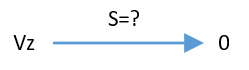$$S=\frac{V^2_z-0^2}{2a_{max}}=\frac{V^2_z}{2a_{max}}$$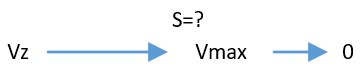$$S=\frac{V^2_z-V_{max}^2}{2a_{1}}+\frac{1}{2}a_{max}t^2$$

*V_max是单位时间内最大加速度能得到的最快速度，即V_max=a_maxt**，a1为V_max到Vz的加速度。

linear_dist = second_ord_lim/sq(p); 

$$linear_{dist}=\frac{a_{max}}{K_p^2}=a_{max}t^2$$$$V_{max}=\frac{a_{max}}{K_p}=a_{max}t$$$$linear_{dist}=V_{max}t$$

|err|<linear_dist时

correction_rate = error*p;

$$correction_{rate} = err*K_p$$

|err|>linear_dist时

linear_dist = second_ord_lim/sq(p);
correction_rate = safe_sqrt(2.0f*second_ord_lim*(error-(linear_dist/2.0f)));

$$correction_{rate} = \sqrt{2a_{max}\times (err-\frac{1}{2}a_{max}t^2)}$$

$$err = a_zt^2 < a_{max}t^2$$

$$correction_{rate} = err*K_p = a_zt^2/t=a_zt=V_z$$

$$err=\frac{1}{2}a_{max}t^2 +\frac{V_z^2}{2a_{max}}>\frac{1}{2}a_{max}t^2 +\frac{V_{max}^2}{2a_{max}}=a_{max}t^2\\ correction_{rate} = \sqrt{2a_{max}\times (err-\frac{1}{2}a_{max}t^2)}=V_z$$

ok,现在很明显了，计算刹车距离的函数，就是为了配合开方控制器，是刹车函数启动的时候，可以是刹车的瞬间，期望速度为当前速度，即可以平滑减速，不会有速度突变。

$$linear_{dist}=\frac{a_{max}}{K_p^2}=a_{max}t^2 \\$$

$$correction_{rate}=a_t^2*K_P= at , a<a_{max}$$

$$err>a_{max}t^2$$

$$err = (2+c)*\frac{1}{2}a_{max}t^2,c>0$$$$correction_{rate}=\sqrt{2a_{max}\times \frac{1}{2}a_{max}t^2(2+c-1)} \\ =\sqrt{2a_{max}\times \frac{1}{2}a_{max}t^2(1+c)}=a_{max}t\sqrt{1+c}$$

$$correction_{rate}= at ,|a|<=|a_{max}| \\ correction_{rate}= a_{max}t\sqrt{1+c} ,|a|>|a_{max}|,c>0$$

correction_{rate} 就是 期望速度 ，与当前速度做差，经过PID ，就得到了期望加速度。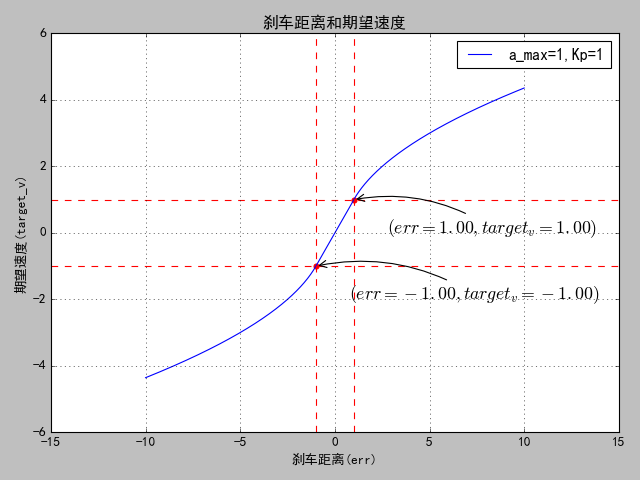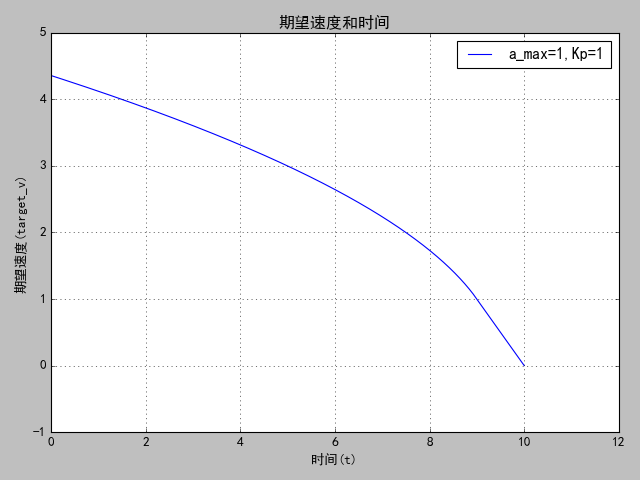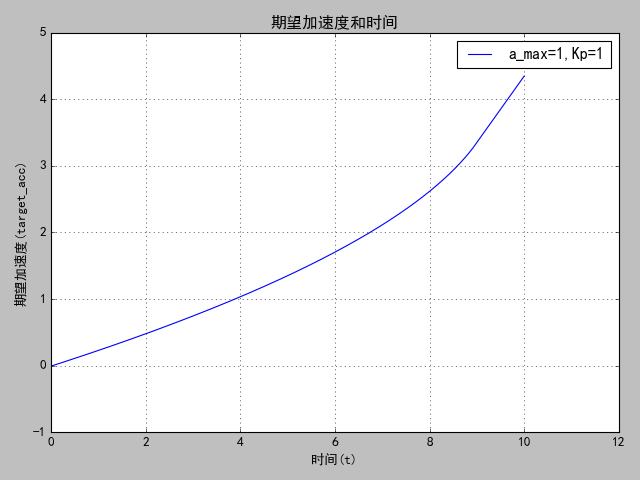1.初始期望加速度为0，飞机的姿态不会突变。

2.加速度缓慢增加，直到等于最大加速度，飞机在刹车过程姿态变化平滑。

get_stopping_point_z

void AC_PosControl::get_stopping_point_z(Vector3f& stopping_point) const
{
const float curr_pos_z = _inav.get_altitude();
float curr_vel_z = _inav.get_velocity_z();
float linear_distance;
float linear_velocity;

if (is_active_z()) {
curr_vel_z += _vel_error.z;
if (_flags.use_desvel_ff_z) {
curr_vel_z -= _vel_desired.z;
}
}

if (_p_pos_z.kP() <= 0.0f || _accel_z_cms <= 0.0f) {
stopping_point.z = curr_pos_z;
return;
}
linear_velocity = _accel_z_cms/_p_pos_z.kP();

if (fabsf(curr_vel_z) < linear_velocity) {
stopping_point.z = curr_pos_z + curr_vel_z/_p_pos_z.kP();
} else {
linear_distance = _accel_z_cms/(2.0f*_p_pos_z.kP()*_p_pos_z.kP());
if (curr_vel_z > 0){
stopping_point.z = curr_pos_z + (linear_distance + curr_vel_z*curr_vel_z/(2.0f*_accel_z_cms));
} else {
stopping_point.z = curr_pos_z - (linear_distance + curr_vel_z*curr_vel_z/(2.0f*_accel_z_cms));
}
}
stopping_point.z = constrain_float(stopping_point.z, curr_pos_z - POSCONTROL_STOPPING_DIST_DOWN_MAX, curr_pos_z + POSCONTROL_STOPPING_DIST_UP_MAX);
}

sqrt_controller

float AC_AttitudeControl::sqrt_controller(float error, float p, float second_ord_lim, float dt)
{
float correction_rate;
if (is_negative(second_ord_lim) || is_zero(second_ord_lim)) {
correction_rate = error*p;
} else if (is_zero(p)) {
if (is_positive(error)) {
correction_rate = safe_sqrt(2.0f*second_ord_lim*(error));
} else if (is_negative(error)) {/
correction_rate = -safe_sqrt(2.0f*second_ord_lim*(-error));
} else {
correction_rate = 0.0f;
}
} else {
float linear_dist = second_ord_lim/sq(p);
if (error > linear_dist) {
correction_rate = safe_sqrt(2.0f*second_ord_lim*(error-(linear_dist/2.0f)));
} else if (error < -linear_dist) {
correction_rate = -safe_sqrt(2.0f*second_ord_lim*(-error-(linear_dist/2.0f)));
} else {
correction_rate = error*p;
}
}
if (!is_zero(dt)) {
return constrain_float(correction_rate, -fabsf(error)/dt, fabsf(error)/dt);
} else {
return correction_rate;
}
}